Question

# What is the marginal probability of rolling a 2 using two six-sided dice? Round your answer...

What is the marginal probability of rolling a 2 using two six-sided dice? Round your answer to three decimal places

What is the probability of rolling something other than double 2s using two six-sided dice? Round your answer to three decimal places

1)

marginal probability of rolling a 2 using two six-sided dice =11/36 (as there are 11 ways such that a 2 can appear on a pair of dice)
2)

probability of rolling something other than double 2s using two six-sided dice =35/36

(as there is only one way out of 36 a double 2 can appear ; therefore number of ways it does not appear are35)

#### Earn Coins

Coins can be redeemed for fabulous gifts.

Similar Homework Help Questions
• ### What is the probability of rolling something other than double 4s using two six-sided dice? Round...

What is the probability of rolling something other than double 4s using two six-sided dice? Round your answer to three decimal places.

• ### A standard pair of six-sided dice is rolled. What is the probability of rolling a sum...

A standard pair of six-sided dice is rolled. What is the probability of rolling a sum less than 5? Express your answer as a fraction or a decimal number rounded to four decimal places.

• ### A standard pair of six-sided dice is rolled. What is the probability of rolling a sum...

A standard pair of six-sided dice is rolled. What is the probability of rolling a sum greater than 9? Express your answer as a fraction or a decimal number rounded to four decimal places.

• ### A standard pair of six-sided dice is rolled. What is the probability of rolling a sum...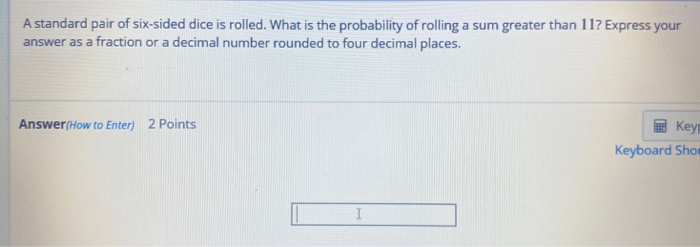A standard pair of six-sided dice is rolled. What is the probability of rolling a sum greater than 117 Express your answer as a fraction or a decimal number rounded to four decimal places. Answer/How to Enter) 2 Points Key Keyboard Shor I

• ### Correct A standard pair of six-sided dice is rolled. What is the probability of rolling a...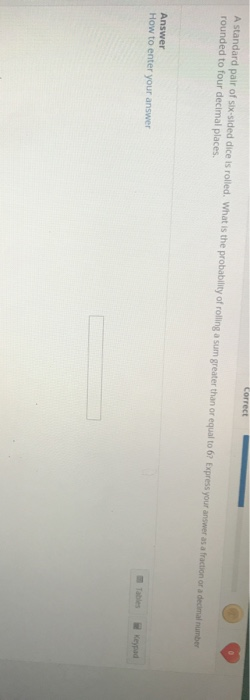Correct A standard pair of six-sided dice is rolled. What is the probability of rolling a sum greater than or equal to 62 Express your answer as a fraction or a decimal number rounded to four decimal places Answer How to enter your answer

• ### What is the probability of rolling a sum of 6 on a standard pair of six-sided...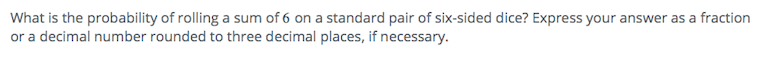What is the probability of rolling a sum of 6 on a standard pair of six-sided dice? Express your answer as a fraction or a decimal number rounded to three decimal places, if necessary.

• ### If two six sided dice are rolled, what is the probability of rolling a 5 on...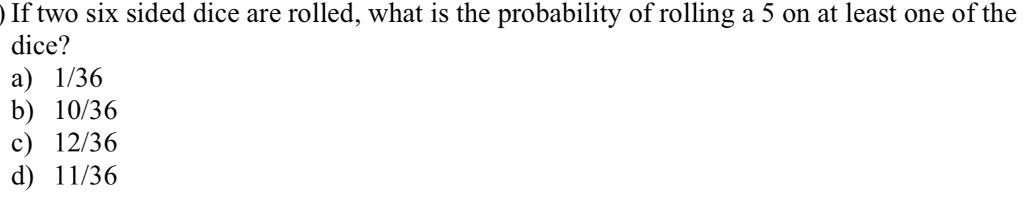If two six sided dice are rolled, what is the probability of rolling a 5 on at least one of the dice? a) I/36 b) 10/36 c) 12/36 d) 11/36

• ### Two fair six-sided dice are rolled. What is the probability that one die shows exactly three...

Two fair six-sided dice are rolled. What is the probability that one die shows exactly three more than the other die (for example, rolling a 1 and 4, or rolling a 6 and a 3)

• ### Using a standard deck of 52 cards and two six-sided dice, what is the probability that...

Using a standard deck of 52 cards and two six-sided dice, what is the probability that you pick or roll the number 2? Exclude the possibility of picking and rolling the number 2.

• ### In the game of "Craps", a roller plays by rolling two six-sided dice. The score for...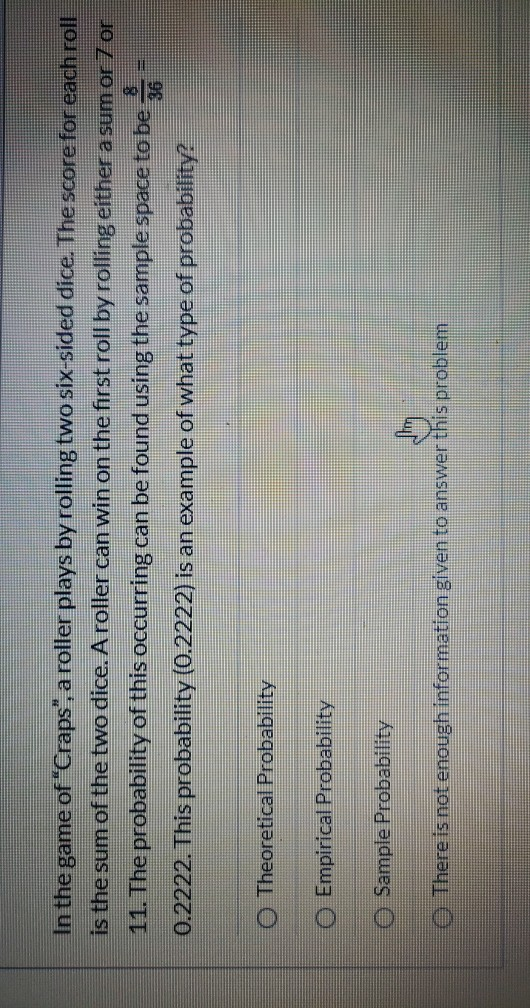In the game of "Craps", a roller plays by rolling two six-sided dice. The score for each roll is the sum of the two dice. A roller can win on the first roll by rolling either a sum or 7 or 11. The probability of this occurring can be found using the sample space to be 0.2222. This probability (0.2222) is an example of what type of probability? O Theoretical Probability O Empirical Probability O Sample Probability There is not...